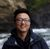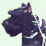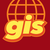611
9
05-06-2022 12:23 AM
Status: Under Consideration
Labels (1)New Contributor II

Working primarily with data in UTM projection, I often need ways to convert UTM coordinates to WGS to create deep links to various online services.

Having to create duplicate datasets, or running python scripts as regular intervals is tedious and time consuming.

And arcade equivalent of the python projectAs method would solve almost all these issues.

Tags (4)byStatus changed to: Under Consideration

We've been discussing this on the development team. If others have different use cases, please feel free to add them to the comments.by

I can't agree more with this. As the largest GIS software company on earth, I was surprised that Arcade doesn't have function like proj4.js built-in.

The solution here works, but still hope to have something more built-in 🙂

Solved: Lat/Long unit conversion with Arcade - Esri Community

RenoI'm looking for similar functionality, my use case is to auto calculate areas in hectares or length in metres for features in a geographic coordinate system.Being able to have a form in a webmap automatically calculate x and y coordinates in local projections systems upon data capture/data edit  via arcade would be hugely advantageous. Having to manually calculate these fields leads to a danger of them not being updated when data is edited.by

It would be extremely helpful to have access to a projection library (e.g., PROJ4JS | Proj4js) in ArcGIS Arcade to support the calculation of coordinates in differing systems (e.g., USNG and Latitude\Longitude). Arcade will only return the XY coordinates in the projection of the dataset.

The only solution is to translate the spherical trig into Arcade

``````// Calculates the latitude of a State Plane coordinate
// Get Colorado State Plane North (2231) coordinates from geometry
var x = Geometry(\$feature).x
var y = Geometry(\$feature).y

// Projection Information
var linear_unit = 0.3048006096012192
var false_easting = 3000000.000316083
var false_northing = 999999.999996
var sp1 = 39.71666666666667
var sp2 = 40.78333333333333
var latitude_of_origin = 39.33333333333334
var semimajor_axis = 6378137.0
var semiminor_axis = 6356752.314140356

return (deg * PI / 180)
}

return (rad * 180) / PI
}

function tVal(t) {
return (Tan((PI / 4) - (t / 2)) / Pow((1 - (ecc * Sin(t))) / (1 + (ecc * Sin(t))), (ecc / 2)))
}

// Convert projection information into radians

// Spherical Trig voodoo
var flat = 1 / (semimajor_axis / (semimajor_axis - semiminor_axis))
var ecc = Sqrt(2 * flat - Pow(flat, 2))
var n = (Log(Cos(sp1R) / Pow((1 - Pow(ecc, 2) * Pow(SIN(sp1R), 2)), 0.5)) - Log(Cos(sp2R) / Pow((1 - Pow(ecc, 2) * Pow(SIN(sp2R), 2)), 0.5))) / (Log(tVal(sp1R)) - Log(tVal(sp2R)))
var f = (Cos(sp1R) / Pow((1 - Pow(ecc, 2) * Pow(SIN(sp1R), 2)), 0.5)) / (n * Pow(tVal(sp1R), n))
var rf = (semimajor_axis * (1 / linear_unit)) * f * Pow(tVal(lorR), n)
var rz = Pow(Pow((x - false_easting), 2) + Pow((rf - (y - false_northing)), 2), 0.5)
var tz = Pow((rz / ((semimajor_axis * (1 / linear_unit)) * f)), (1 / n))

var latTR = (PI / 2) - (2 * Atan(tz))
var lat1R = (PI / 2) - (2 * Atan((tz * Pow((1 - (ecc * Sin(latTR))) / (1 + (ecc * Sin(latTR))), (ecc / 2)))))
var lat2R = (PI / 2) - (2 * Atan((tz * Pow((1 - (ecc * Sin(lat1R))) / (1 + (ecc * Sin(lat1R))), (ecc / 2)))))
var lat3R = (PI / 2) - (2 * Atan((tz * Pow((1 - (ecc * Sin(lat2R))) / (1 + (ecc * Sin(lat2R))), (ecc / 2)))))
var lat4R = (PI / 2) - (2 * Atan((tz * Pow((1 - (ecc * Sin(lat3R))) / (1 + (ecc * Sin(lat3R))), (ecc / 2)))))
var latFR = (PI / 2) - (2 * Atan((tz * Pow((1 - (ecc * Sin(lat4R))) / (1 + (ecc * Sin(lat4R))), (ecc / 2)))))

// Latitude values in degrees
return latitude;``````+1 from me. This would be useful for data interoperability.+1 from me! My whole organization is longing for this!I also have a need for this based on a recent request to convert from State Plane to Decimal Degrees in an attribute ruleMy team is constantly asked to show a point's lat/lon as a standard attribute, this idea would simplify those requests and ease maintenance.